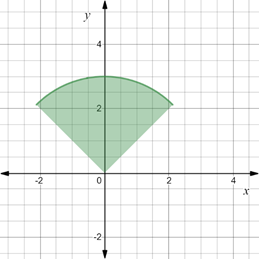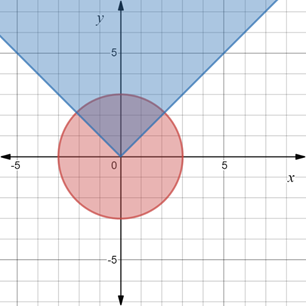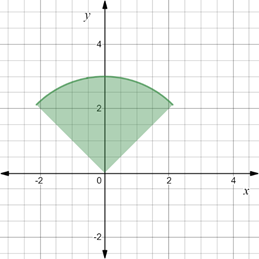# The area common to the regions formed by the inequalities x 2 + y 2 ≤ 9 and y ≥ | x | . Also sketch the region that satisfy both the inequalities.### Precalculus: Mathematics for Calcu...

6th Edition
Stewart + 5 others
Publisher: Cengage Learning
ISBN: 9780840068071### Precalculus: Mathematics for Calcu...

6th Edition
Stewart + 5 others
Publisher: Cengage Learning
ISBN: 9780840068071

#### Solutions

Chapter 1.8, Problem 112E
To determine

## To calculate: The area common to the regions formed by the inequalities x2+y2≤9 and y≥|x| . Also sketch the region that satisfy both the inequalities.

Expert Solution

The area of the region is A=9π4 sq. units . The graph of the region is provided below,### Explanation of Solution

Given information:

The inequalities x2+y29 and y|x| .

Formula used:

The standard form of the equation of the circle is (xh)2+(yk)2=r2 , where (h,k) denote the center of the circle and r denote the radius.

Calculation:

Consider the provided inequalities x2+y29 and y|x| .

Rewrite the inequality x2+y29 as an equation of circle, x2+y2=32 .

And (x0)2+(y0)2=32 .

Recall that the standard form of the equation of the circle is (xh)2+(yk)2=r2 , where (h,k) denote the center of the circle and r denote the radius.

Compare, (h,k) and (0,0) also radius r is 3.

Therefore, inequality is a circle with center at (0,0) and radius 3.

Now, the inequality contains all point (x,y) in the coordinate plane that satisfy x2+y29 .

Also, y|x| is yxy . That is plot the equation of lines y=x and y=x . Shade the region that lie between the lines.

Red shaded region represents x2+y29 and the blue shaded region represents y|x|Now, the region common to the inequalities is shaded by green color which is a sector with included angle as θ=π2 and radius as 3 units.The area of circular sector is expressed as A=12r2θ .

Substitute r as 3 and θ=π2 in the above expression,

A=12r2θ=12(3)2π2=9π4

Thus, the area of the region is A=9π4 sq. units .

### Have a homework question?

Subscribe to bartleby learn! Ask subject matter experts 30 homework questions each month. Plus, you’ll have access to millions of step-by-step textbook answers!